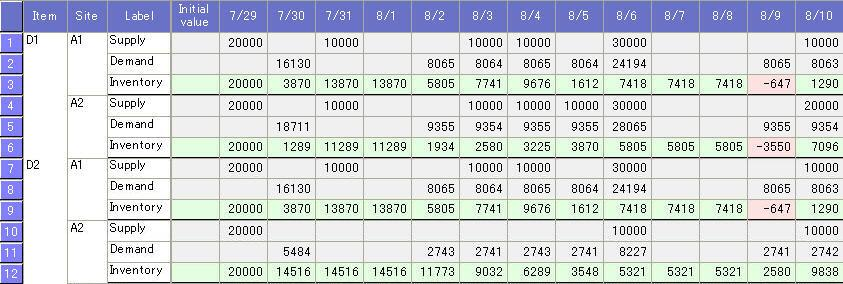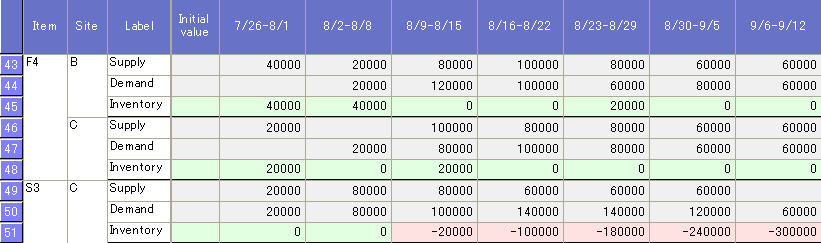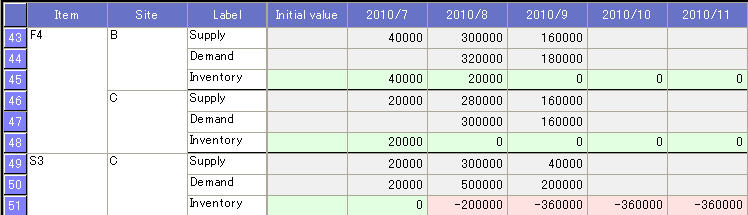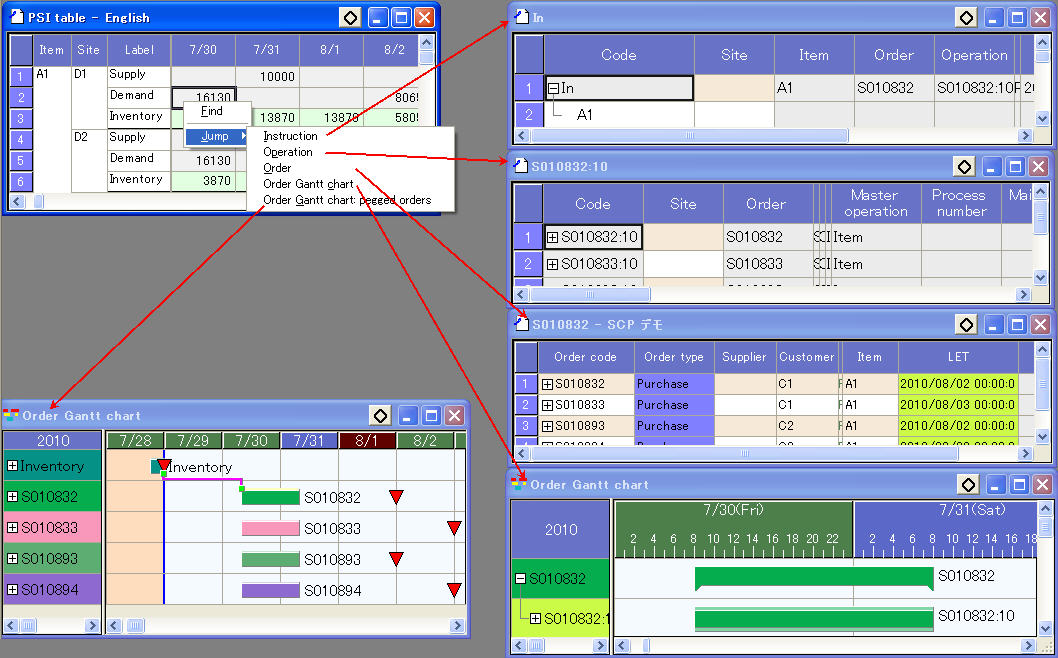## PSI(Asprova SCP)

PSI(Asprova SCP) is a table which summarize "Site, Item", "Item, Site" or "Supply, Demand" daily, weekly or monthly. The inventory quantity is displayed in the cell. The background color will be different according to specified condition to display the inventory fluctuation obviously.

Select "Table view/PSI" from main menu to open PSI table.

Default style

There are some default styles in PSI(Asprova SCP) as follows.

Site, item

The site of the item is grouped by item in vertical axis. The time unit of the horizontal axis is one day. The daily supply, demand and inventory of each item in each site is displayed in the cells.

There are 3 objects displayed in each group in vertical axis

Supply: Summary of output instruction quantity on this day

Demand: Summary of input instruction quantity on this day

Inventory: Inventory quantity at the end of this day

In case of inventory, the initial value is displayed. The initial value is the inventory quantity at the earliest start time.
The inventory quantity is fluctuated based on the initial value according to the supply and demand quantity. If the inventory quantity is below zero, the background color of the cell is marked with red, otherwise it will be marked with green.Item, site

The item of the site is grouped by site in vertical axis. The time unit of the horizontal axis is one day. The daily supply, demand and inventory of each item in each site is displayed in the cells.

There are 3 objects displayed in each group in vertical axis

Supply: Summary of output instruction quantity on this day

Demand: Summary of input instruction quantity on this day

Inventory: Inventory quantity at the end of this day

In case of inventory, the initial value is displayed. The initial value is the inventory quantity at the earliest start time.
The inventory quantity is fluctuated based on the initial value according to the supply and demand quantity. If the inventory quantity is below zero, the background color is marked with red, otherwise it will be marked with green.Weekly summary

The site of item is grouped by item in vertical axis. The time unit of the horizontal axis is one week. The weekly supply, demand and inventory of each item in each site is displayed in the cells.

There are 3 objects displayed in each group in vertical axis

Supply: Summary of output instruction quantity on this day

Demand: Summary of input instruction quantity on this day

Inventory: Inventory quantity at the end of this day

In case of inventory, the initial value is displayed. The initial value is the inventory quantity at the earliest start time.
The inventory quantity is fluctuated based on the initial value according to the supply and demand quantity. If the inventory quantity is below zero, the background color is marked with red, otherwise it will be marked with green.Weekly summary

The site of item is grouped by item in vertical axis. The time unit of the horizontal axis is one month. The monthly supply, demand and inventory of each item in each site is displayed in the cells.

There are 3 objects displayed in each group in vertical axis

Supply: Summary of output instruction quantity on this day

Demand: Summary of input instruction quantity on this day

Inventory: Inventory quantity at the end of this day

In case of inventory, the initial value is displayed. The initial value is the inventory quantity at the earliest start time.
The inventory quantity is fluctuated based on the initial value according to the supply and demand quantity. If the inventory quantity is below zero, the background color is marked with red, otherwise it will be marked with green.Because the input instruction and output instruction of operations are used in PSI table, the assigned status of operations is displayed in the table except operations with "Unassigned" flag. The use instructions are all deleted after "All unassign". Then the PSI table will be null.

Jump

Right click on the cell with value, a popup menu will display. If you select a menu, it will jump to the input instruction table or output instruction table related to the value. In addition, it will also jump to operation or order table or graph related to the value.